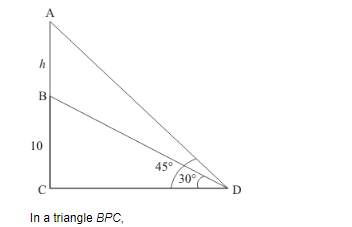# From a point P on the ground the angle of elevation of a 10 m tall building is 30°.

Question:

From a point P on the ground the angle of elevation of a 10 m tall building is 30°. A flag is hoisted at the top of the building and the angle of elevation of the top of the flag-staff from P is 45°. Find the length of the flag-staff and the distance of the building from the point P. (Take $\sqrt{3}=1.732$ ).

Solution:

Letbe the flag of lengthm on the building.

We assume that $B C=10, C P=y$ and $\angle A P C=45^{\circ}, \angle B P C=30^{\circ}$

Now we have to find height of flag-staff and distance of the point P from the building

The corresponding figure is as follows$\Rightarrow \quad \tan P=\frac{B C}{C P}$

$\Rightarrow \quad \tan 30^{\circ}=\frac{B C}{C P}$

$\Rightarrow \quad \frac{1}{\sqrt{3}}=\frac{10}{y}$

$\Rightarrow \quad y=10 \sqrt{3}$

$\Rightarrow \quad y=17.32$

Again in a triangle ACP,

$\Rightarrow \quad \tan P=\frac{A B+B C}{C P}$

$\Rightarrow \quad \tan 45^{\circ}=\frac{h+10}{y}$

$\Rightarrow \quad \mathrm{I}=\frac{h+10}{17.32}$

$\Rightarrow \quad h=10-17.32$

$\Rightarrow \quad h=7.32$

Hence the length is $17.32 \mathrm{~m}$ and distance is $7.32 \mathrm{~m}$.